# One more approach to optimize neural networks

Talking about Neural Architecture Search and own algorithm for optimizing neural network hyperparameters

In the last decade, neural network-based solutions have become extremely popular. At the same time, deep learning is quite a complex field, requiring high theoretical knowledge from experts. The industry needs quite a lot of these specialists, but now there are not enough of them to satisfy the request. With this gap between supply and demand, special tools are emerging. Let’s call these tools “automated tools”.

Such tools are appearing in other industries as well. For example, special machines allow automating car assembling, and irrigation systems allow automating agriculture. As a result of the technology, fewer specialists are needed to assemble cars and irrigation.

The same processes are observed in modern “Data Science”. Various Automated machine learning (AutoML) frameworks and libraries appear. All of them allow us to automate the process of model identification. For classical machine learning tasks (classification, regression) on tabular data, the H2OTPOTAutoGluonLightAutoML, or FEDOT frameworks might be used. Such libraries are called AutoML frameworks. However, the field of identification of deep learning models has been taken up by technologies of a slightly different nature. The so-called Neural Architecture Search (NAS) is commonly used here.

Generally speaking, all NAS algorithms belong to the AutoML category. However, there is a significant difference in approaches because of the specifics of neural networks. Firstly, neural networks tend to have much more hyperparameters than classical machine learning algorithms (imagine ResNet and a linear regression model, for example, and compare them). Secondly, neural networks usually require much more resources for computation. This is a big problem, which limits the set of algorithms for optimization, which can be used for neural networks.

Disclaimer: AutoML and NAS are great! More and more routine things are being done with software, leaving more time for experts to conduct interesting experiments. I am involved in the development of the AutoML tool and believe that such instruments are the next step in the evolution of Data Science.

## Neural Architecture Search

A neural network is a complex model. It consists of a large number of components (hyperparameters). An expert needs to determine the topology of the neural network, how many layers, how many neurons, what activation functions, batch size, and more before starting training the model. It is worth remembering that a neural network requires a lot of resources to train. So, it is too complicated to fully enumerate all these hyperparameters using grid search.

Algorithms instead of an expert can choose a model. Such algorithms are, for example, libraries AutoKerasNNIENAS in PyTorch, and others. Some approaches allow quickly implementing such optimizers yourself for simple architectures. For example, based on the optuna optimization library — examples. On the other hand, there are also intriguing academic frameworks that look for an optimal neural network based on evolutionary algorithms — nas-fedot and NAS-object-recognition (the development of these modules is handled by colleagues from our lab :)).

The topic is really popular, so there are many tools, I have listed here a very small part of them. But if you are interested in the topic, you can start with them. Many more solutions exist in the form of concepts, for example, in scientific papers in journals and conference proceedings. Use the keywords “neural architecture search” to search, and you will find many articles (I promise) (Figure 1).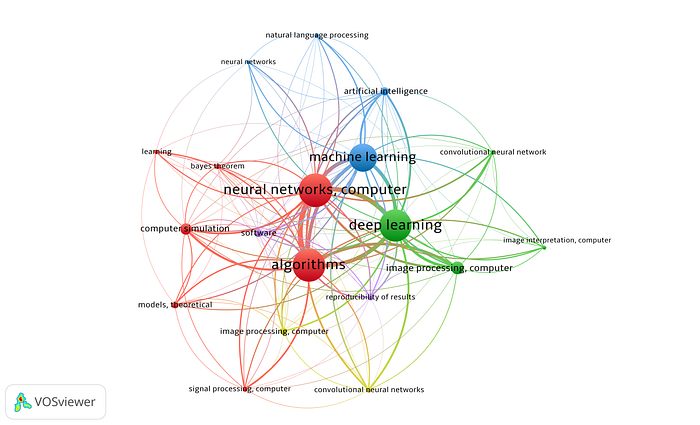Figure 1. Topics with “NAS” in scientific papers for the last five years (since 2017). Information retrieved from https://www.lens.org/ (image by author)

## Another approach?

There was cited a small part of all the developments that are taking place in the field of NAS. And yes, I want to introduce another one. The motivation for this is the assumption: I believe that increasing the number of neurons and layers is a reliable way to increase the accuracy of the algorithm. But at the same time, it noticeably increases the “computational cost” of training the neural network, as well as the size of the model. In this case, the search space becomes too large to find something really optimal also. Perhaps if we limit to given neural network topology and optimize the activation functions and other hyperparameters (without changing the number of layers and neurons) it will work just as well.

So, let’s try to make an algorithm that optimizes some hyperparameters of the neural network. The number of neurons and the number of layers do not change during the optimization. This makes it possible to reduce the search space.

## “The most important thing is the logo”

Honestly, it’s quite important. At least for me. The algorithm and the concept are nice, but the experience of developing the product is important also. And the experience consists of exterior attributes, such as the design of the repository, documentation, and related materials.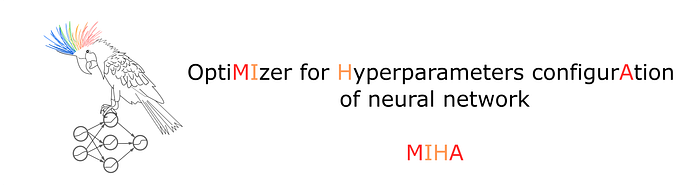Figure 2. The logo for the module that is being developed (image by author)

Actually, the concept is more important than the logo

But the concept is vital too. So let’s explore the core idea of the algorithm.

The first specific feature I encountered before starting to implement an algorithm is the high computational cost of NAS algorithms. Indeed, to train several neural networks for a fairly large number of epochs is time-consuming. So it was decided to train just one neural network, but to change some hyperparameters during training. At certain moments a small number of neural networks with alternative configurations of hyperparameters are generated. To determine how successful the proposed changes have been, it is suggested to use the increase in the metric over several epochs (Figure 3).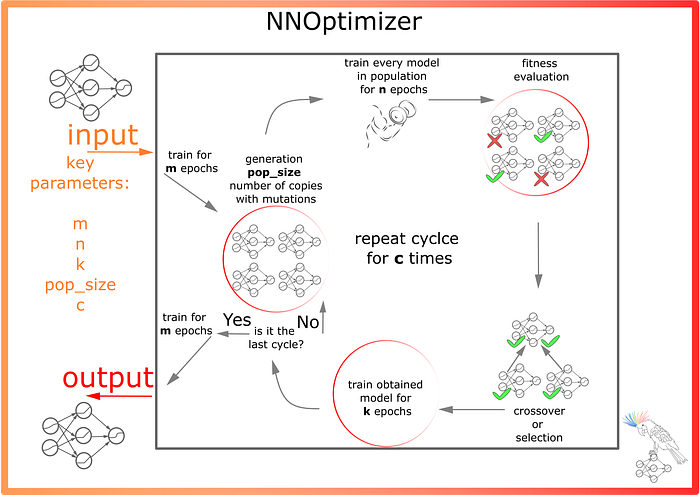Figure 3. The main idea of the optimization algorithm (image by author)

The main hyperparameters are m — number of epochs for initial and final training, pop_size — the number of generated neural networks with alternative hyperparameter configurations, n — the number of epochs devoted to the training of each “alternative neural network”, k — number of epochs for fixing training of the intermediate neural network after crossover or selection, — number of cycles with population generation and changes. As can be seen from the names of the hyperparameters, terms from the field of evolutionary computation are used: the set of alternative neural networks is called a population, the procedure for evaluating the effectiveness of models is called a fitness evaluation. A change in hyperparameters — a mutation. However, the proposed approach is not truly evolutionary (although it is quite similar to it).

The optimization process is shown in the animation below. For convenience, each intermediate neural network is serialized in a zip archive. If necessary, it is possible to specify the flag so that the folder with serialized models is deleted after the end of the computation.

As can be seen from the animation, a single neural network with an initial configuration of hyperparameters is trained first. Then several alternative models are generated, in each of which the hyperparameter is replaced. The current prototype is capable of making the following changes:

• Change activation function in a randomly selected layer;
• Change the batch size;

Not much, but even with this approach the search space is quite large.

The most successful replacement is selected based on fitness evaluation:

• The value of the fitness function depends on the absolute value of the last received value of the loss function for a neural network;
• The value of the fitness function depends on the rate of learning (how fast the loss function changes from epoch to epoch).

The most successful model is trained over several epochs. And then the cycle with replacement is repeated.

## Technical details

The module is based on three main parts: optimizer (model), evolutionary operators (evolutionary), and logging system (Figure 4).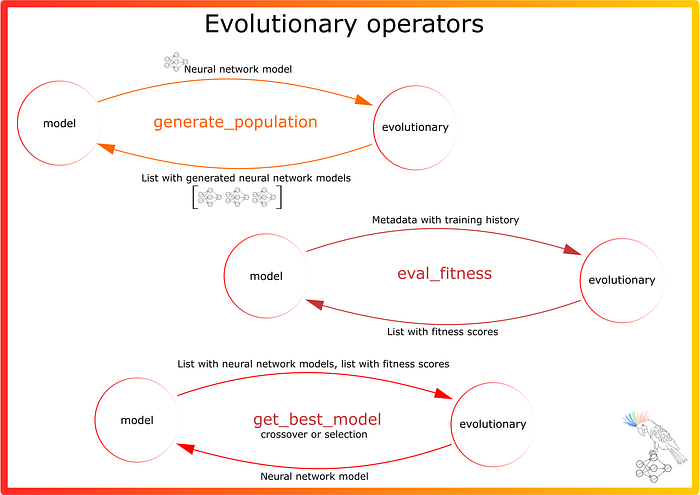Figure 4. The main ways of interaction between the different parts (classes and methods) of the program (image by author)

I found it especially valuable to implement a logging system. In the process of optimization a lot of important information is stored (Figure 5).

In addition to the information, the models are also stored. It is worth noting that they are all already trained, and represent ready-to-use models. To make it easier to understand the names of the files, the following picture is prepared (Figure 6).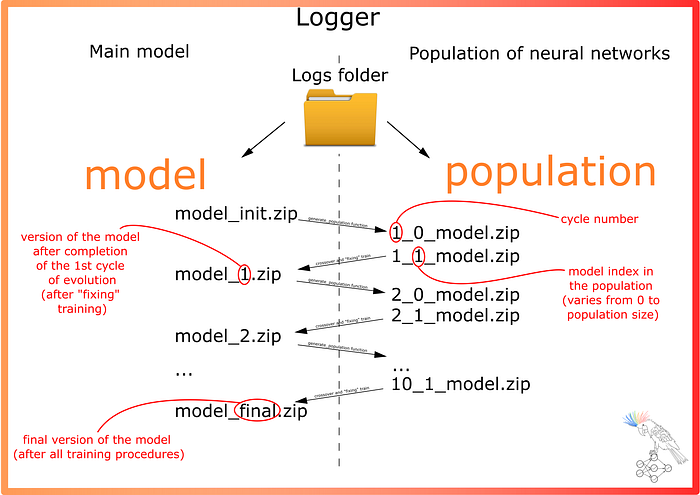Figure 6. Archive names with serialized neural networks (image by author)

As it was written earlier, all these models are saved in a folder. If it is required that only the final model remains after all the calculations, it can be specified that the folder is deleted after the experiment. Thus, based on the experiment with running the model, the following learning history can be obtained: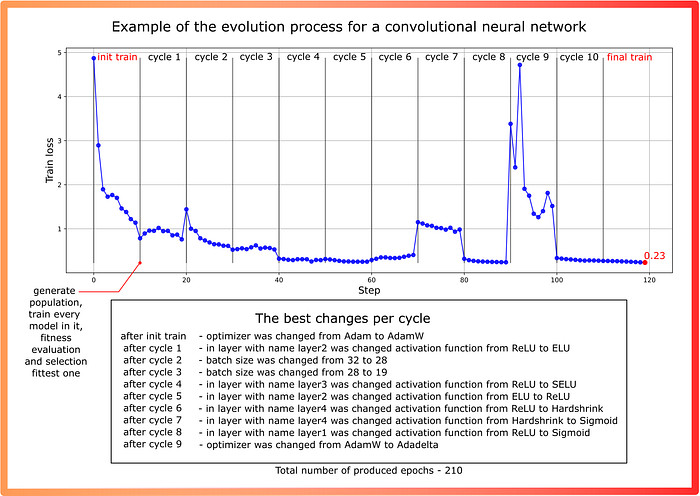Figure 7. Example of one of the test launches of the algorithm (image by author)

## Experiments

To find out how effective the algorithm is, it was decided to conduct several experiments on different tasks for different neural network architectures:

• Applications of the algorithm for Feedforward neural network (FNN) optimization. Task — multi-class classification of the MNIST dataset and comparison with the Optuna framework (see example);
• Applications of the algorithm for Convolutional neural network (CNN) optimization. Task — gap-filling in remote sensing products (The effectiveness of the algorithm is compared with init neural network training without hyperparameters search).

In this jupyter notebook, a comparison was made with Optuna in terms of accuracy metrics. The optuna optimizer increases the number of layers in the neural network, while MIHA only changes the activation functions, batch size, and optimization algorithm. The following metrics were obtained from the results of the experiments on the test sample: MIHA — 0.974, Optuna — 0.976 (the results are almost the same).